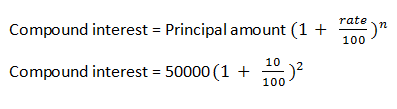# Difference Between Interest Rate and Cash Rate

The word interest rate is used to get heard to everyone whether one knows more or less about the bank. Because the word exists before the bank doesn't exist. But cash rate you might or not heard. Both this rates are types of interest rates and used in banking.

### Interest rate

• An interest rate is a rate at which a charge is either received or paid on saving or borrowed funds.
• An Interest rate is the amount of interest due for a certain period on the proportion of the amount borrowed, lent or deposited.
• In interest rate, a percentage charges on saved or borrowed funds.
• An interest rate charged by the bank or by other rate charges to borrow its money, or the rate payable by a bank to its client for depositing money.
• An interest rate may be calculated monthly quarterly or annually.

### How to calculate:

There are two ways to calculate interest rate

1) Simple interest
FormulaExample:
An amount of RS 50000 is borrowed from a bank at the rate of 10 percent per annum for 2 years at simple interest.Simple interest = 20000

2) Compound interest
FormulaExample: An amount of RS 50000 is borrowed from a bank at the rate of 10 percent per annum for 2 years at compound interest.=60,500
Amount = Compound interest – principal
= 60,500 – 50,000
= 10,500

### Cash rate

• When a commercial bank borrows funds from the central bank, it is called a cash rate.
• In India, the cash rate is referred as a bank rate but the meaning of both are same, used in different countries.
• A cash rate is also known as the overnight money market interest rate.
• A cash rate is paid by the commercial banks on the borrowed funds from the central bank.
• A cash rate has an effect on the interest rate because as if the cash rate increases or decreases, it automatically affects an interest rate.
• The main purpose of increase or decrease in cash rate is to regulate the financial system to run the economy properly.
• A cash rate increases or decreases just in basis points.

### Difference between interest rate and cash rate

Basis Interest rate Cash rate
Meaning An interest rate is a rate at which a charge is either received or paid on saving or borrowed funds. When a commercial bank borrows funds from the central bank, it is called a cash rate.
Managed by An interest rate is managed by the banks. A cash rate is managed by the central authority or bank.
Effect on each other An interest has no effect on cash rate. A cash rate has an effect on the interest rate.
Effect on economy An interest rate directly affects the economy. A cash rate indirectly affects the economy.
Affected by An interest rate is affected by external factors like inflation, government policy etc. A cash rate is generally affected by the internal factors.#### What's trending in BankExamsToday

Smart Prep Kit for Banking Exams by Ramandeep Singh - Download here